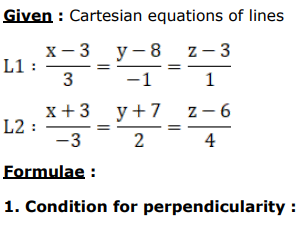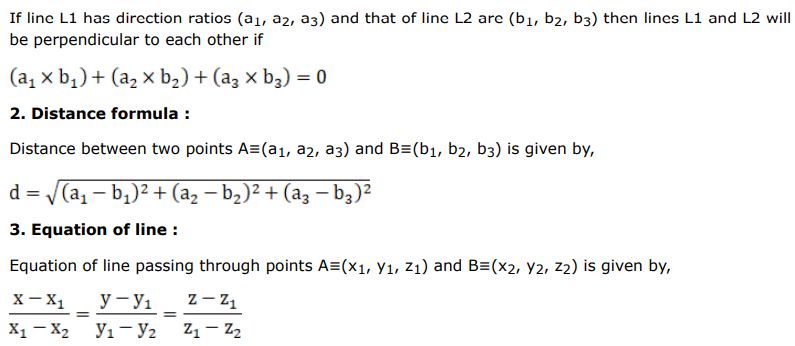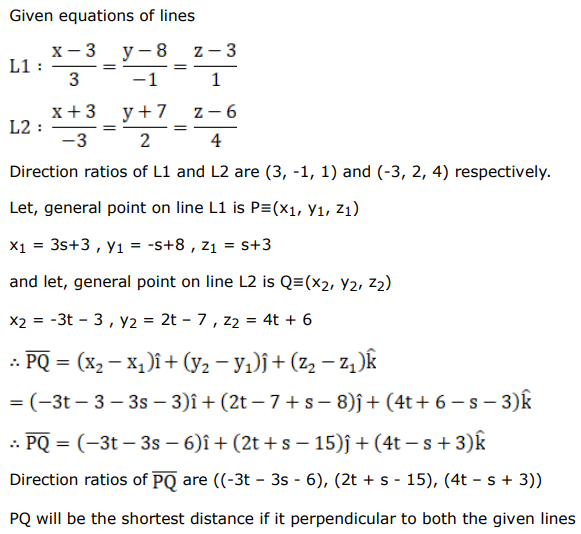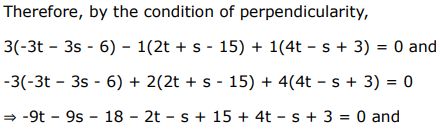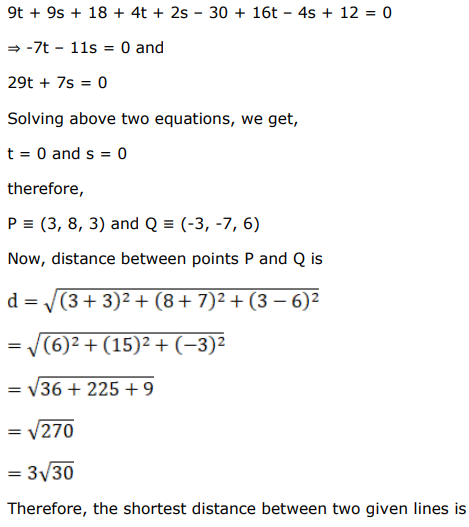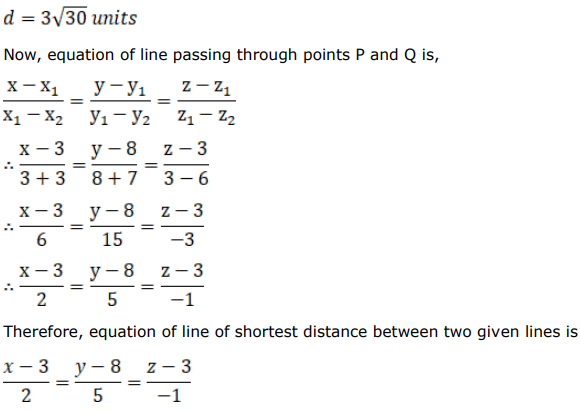# Find the length and the equations of the line of shortest distance between

Question:

Find the length and the equations of the line of shortest distance between the lines given by:

$\frac{x-3}{3}=\frac{y-8}{-1}=z-3$ and $\frac{x+3}{-3}=\frac{y+7}{2}=\frac{z-6}{4} .$

Solution: